## 1. Overview

In this tutorial, we’ll present XOR-linked lists. We’ll start by giving a quick reminder of the single-linked list structure. Then, we’ll move to discuss how are the double-linked lists formed and built.

After that, we’ll describe the structure of XOR-linked lists and how to work with them. Finally, we summarize the advantages and disadvantages of using XOR-linked lists.

The idea of single-linked lists is to store multiple items, one after the other, while maintaining a pointer to the first element. From the first element, we can move to reach the rest of the list. Let’s discuss the structure of a single item and then check how to full list looks.

### 2.1. Item Structure

Take a look at the following shape that shows the structure of a single item in a single-linked list: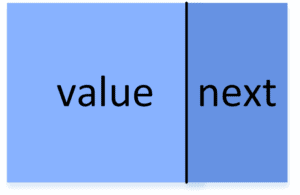Each item consists of two parts. The first part is the, which has the actual content of the current item. The second part is a pointer called, and it points to the next item in the list.

### 2.2. List Structure

The full list contains multiple items. The following figure shows an example of a single-linked list: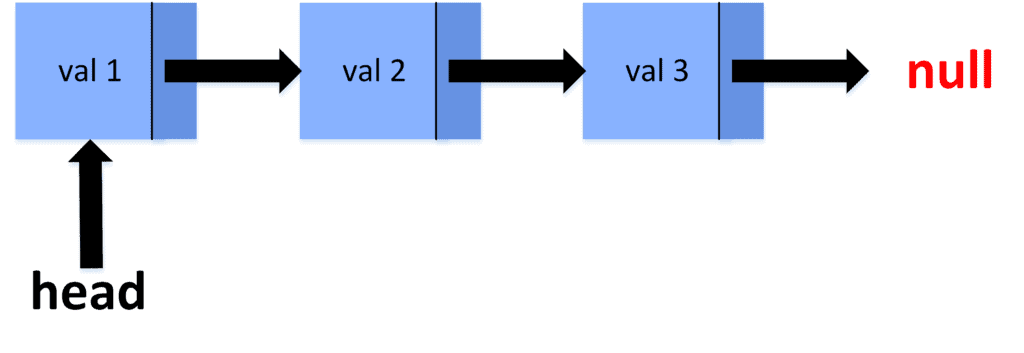As we can see, the list starts with an item that represents the first one in the list. This item is held with a pointer called, necessary to maintain access to the list. Each item from the second one on is maintained by the pointerthat is in the previous item.

It’s important to understand that the pointeris actually an address variable that contains the memory address of the next item.

Note that in single-linked lists, we can start from the, and move forward, accessing items one after the other. However, once we reach an item, we cannot go back to the previous item unless we have a pointer to it stored somewhere else.

The main difference is that double-linked lists contain a pointer to the previous item. Let’s also take a look at the item structure and then see how the list is formed.

### 3.1. Item Structure

Let’s take a look at the following figure that shows the structure of each item in double-linked lists: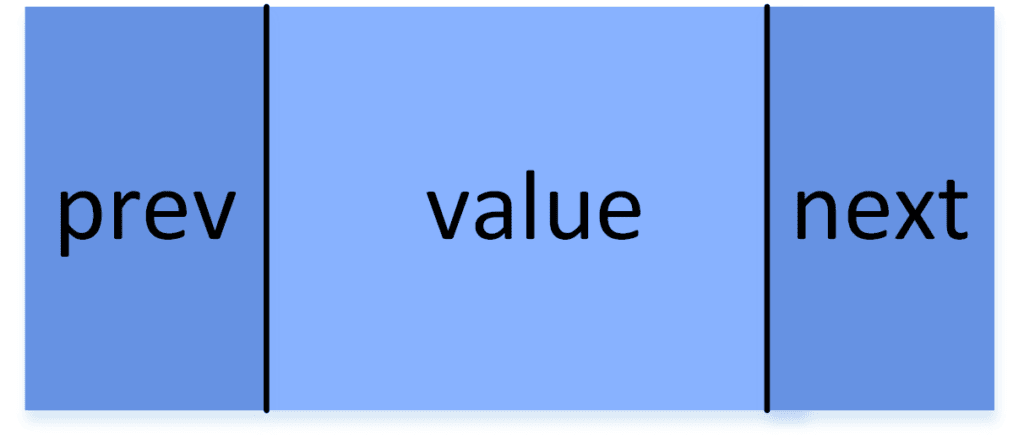Each item has the information stored inside it under the part called. Besides that, it has two pointers (memory addresses) calledand. They hold the memory address of the next and previous items, respectively.

### 3.2. List Structure

Consider the following example of a double-linked list: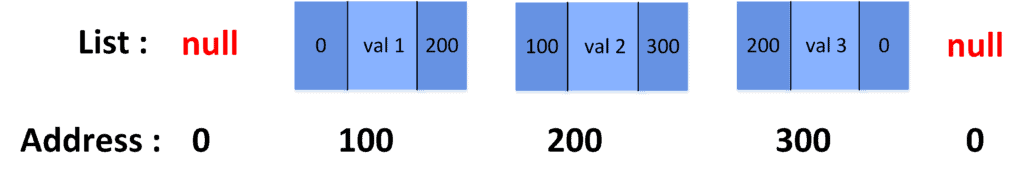This example is more detailed than the one in the single-linked list section. The list consists of 3 items at addresses 100, 200, and 300. As we can see, each item stores the memory address of the next and previous items.

The first item in the list is called, while the last is called. We store their memory addresses in two-pointers. Thus, the value ofwill be 100, while the value ofis 300.

The benefit of having a double-linked list is that, from each item, we can move to either the previous or the next one. However, the drawback is that we had to add an additional memory address variable to our item structure, which can be more memory-consuming.

The idea behind XOR-linked lists is to combine the advantages of both single and double lists. Therefore, we would like to move forward and backwards along the list but without having to store two memory addresses in each item. Let’s see how we can achieve this.

### 4.1. Item Structure

The following figure shows what each item inside XOR-linked lists consists of:Similarly to a single-linked list, the item contains the, and a single memory address. However, the memory address in XOR-linked lists is the logical XOR operator between the address of the previous and next items in the list. The symbolcorresponds to the logical XOR operation.

### 4.2. List Structure

Let’s take a look at how XOR-linked lists look: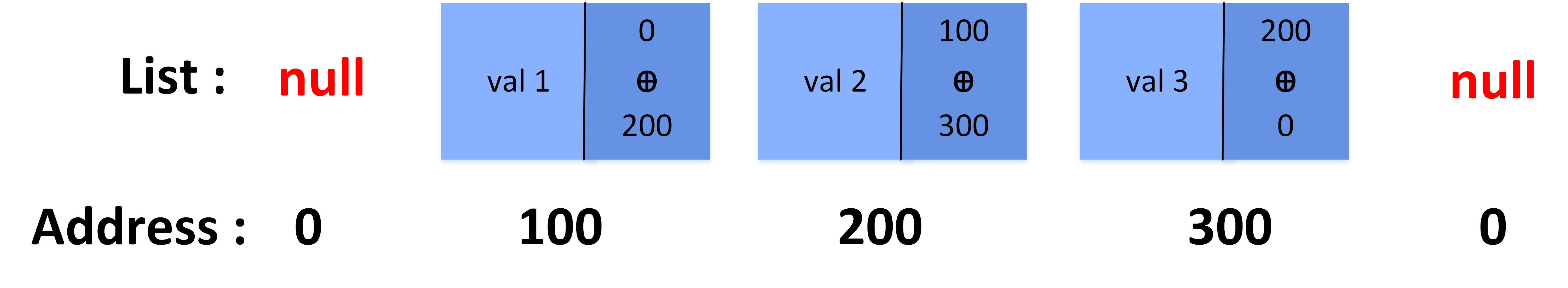As we can see, each element contains a single memory address. Let’s call it, which is short for previous XOR next. This memory address doesn’t point to a specific item. Instead, it contains the logical XOR operation between the memory address of the previous and next elements.

### 4.3. The Reason for Using XOR

The main reason behind using the logical XOR operation is the following formula:Thus, if any value is added an even number of times to XOR operations, then this value is removed from the result.

We can use the previous formula to traverse the XOR-linked list forward and backwards.

### 4.4. Traversing the List Forward

When traversing the list forward, it’s important to always keep in hand the memory address of the previous item. Then, we can calculate the address of the next item using the formula:Sincecontains the value of, adding thevalue one more time will exclude it from the result. Thus, we get the address of the next item.

### 4.5. Traversing the List Backward

When Traversing the list backwards, we need to keep the address of the next item. To calculate the address of the previous item, we use the following formula:Similarly to the previous equation, sincecontains the value of, adding theone more time will exclude it from the result. Thus, we find the address of the previous item.

The main advantage of using XOR-linked lists is that we can move forward and backwards along the list. Thus, we achieve the features of double-linked lists storing only one memory address in each item. As a result, we reduce memory usage while simulating the behaviour of double-linked lists.

On the other hand, using XOR-linked lists has some disadvantages. First, debugging the program becomes much harder because we don’t store the actual addresses anymore inside the item.

Second, the code becomes more complex and harder to understand because of all the XOR operations needed to calculate the address of the previous and next items. Third, garbage collection is difficult because we don’t store any proper addresses.

Therefore, we recommend using XOR-linked lists only when memory usage is becoming really high, and we don’t mind the additional complexities that come from using them.

## 6. Conclusion

In this article, we explained XOR-linked lists. We started by giving quick reminders of single and double-linked lists. Then, we explained the concept of XOR-linked lists and how to traverse them forward and backwards. In the end, we summarised the advantages and disadvantages of using such lists.